# Test: Probability & Statistics- 2

## 30 Questions MCQ Test Topicwise Question Bank for Electrical Engineering | Test: Probability & Statistics- 2

Description
Attempt Test: Probability & Statistics- 2 | 30 questions in 90 minutes | Mock test for GATE preparation | Free important questions MCQ to study Topicwise Question Bank for Electrical Engineering for GATE Exam | Download free PDF with solutions
QUESTION: 1

### A probability density function is of the form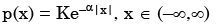The value of K is

Solution:

As (x) is a probability density function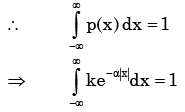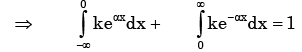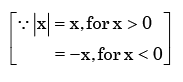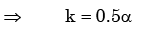QUESTION: 2

### If E denotes expectation, the variance of a random variable X is given by

Solution:

Varience of X = E{( X − m)2 }, m= mean of the distribution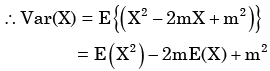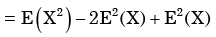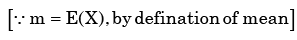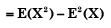QUESTION: 3

### Px(x) = M exp(–2|x|) – N exp(–3 |x|) is the probability density function for the real random variable X, over the entire x axis. M and N are both positive real numbers. The equation relating M and N is

Solution:

Given Px (x ) is the probability density function for the random variable X.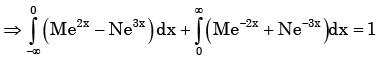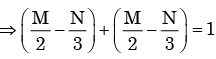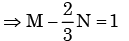QUESTION: 4

A fair coin is tossed independently four times. The probability of the event “the number of time heads shown up is more than the number of times tails shown up” is

Solution:

Here we have to find

P(H, H, H, T) + P(H, H, H, H)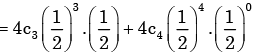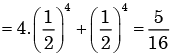QUESTION: 5

The standard deviation of a uniformly distributed random variable between 0 and 1 is

Solution: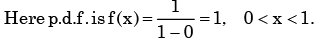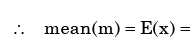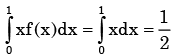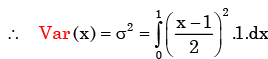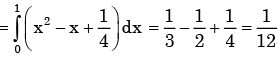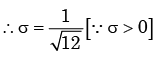QUESTION: 6

The probability of a defective piece being produced in a manufacturing process is 0.01. The probability that out of 5 successive pieces, only one is defective, is

Solution:

The required probability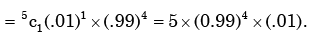QUESTION: 7

An unbiased coin is tossed three times. The probability that the head turns up in exactly two cases is

Solution:

Required probability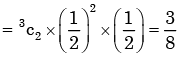QUESTION: 8

Manish has to travel from A to D changing buses at stops B and C enroute. The maximum waiting time at either stop can be 8 minutes each, but any time of waiting up to 8 minutes is equally likely at both places. He can afford up to 13 minutes of total waiting time if he is to arrive at D on time. What is the probability that Manish will arrive late at D?

Solution:
QUESTION: 9

From a pack of regular from a playing cards, two cards are drawn at random. What is the probability that both cards will be Kings, if first card in NOT replaced

Solution:

Here sample space S = 52

∴The probability  of drawing both cards are king without replacement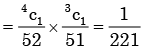QUESTION: 10

A single die is thrown twice. What is the probability that the sum is neither 8 nor 9?

Solution:

Here sample space = 36

Total No. of way in which sum is either 8 or 9  are

(2,6), (3,5),(3,6), (4,4), (4,5), (5,3), (5,4), (6,2), (6,3)

So  probability of getting sum 8 or 9 = 9/36

So the probability of not getting sum 8 or  9 = 1-1/4 = 3/4

QUESTION: 11

A coin is tossed 4 times. What is the probability of getting heads exactly 3 times?

Solution:

Probability of getting exactly three heads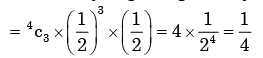QUESTION: 12

A box contains 2 washers, 3 nuts and 4 bolts. Items are drawn from the box at random one at a time without replacement. The probability of drawing 2 washers first followed by 3 nuts and subsequently the 4 bolts is

Solution:

Here sample space =  9

The required probability of drawing 2 washers, 3 nuts and 4 bolts respectively  without replac ement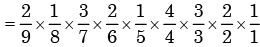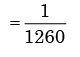QUESTION: 13

Analysis of variance is concerned with:

Solution:

Analysis of variance is used in comparing two or more populations, e.g. Different types of manures for yelding a single crop.

QUESTION: 14

A regression model is used to express a variable Y as a function of another variable X. This implies that

Solution:
QUESTION: 15

A class of first year B. Tech. Students is composed of four batches A, B, C and D, each consisting of 30 students. It is found that the sessional marks of students in Engineering Drawing in batch C have a mean of 6.6 and standard deviation of 2.3. The mean and standard deviation of the marks for the entire class are 5.5 and 4.2, respectively. It is decided by the course instructor to normalize the marks of the students of all batches to have the same mean and standard deviation as that of the entire class. Due to this, the marks of a student in batch C are changed from 8.5 to

Solution:

Let mean and stander deviation of batch C be μc and σc respectively and mean  and standard deviation of entire class of 1st year students be μ and σ  respectively.

Given μ= 6.6 and σ = 2.3

and μ= 5.5 and σ = 4.2

In order to normalize batch C to entire class, the normalized score must be equated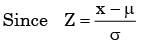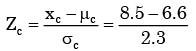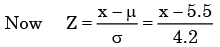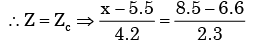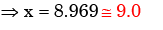QUESTION: 16

A hydraulic structure has four gates which operate independently. The probability of failure off each gate is 0.2. Given that gate 1 has failed, the probability that both gates 2 and 3 will fail is

Solution:

P(gate to and gate 3/gate 1 failed)

= P(gate2 and gate 3)

[ ∴ all three gates are independent corrosponding to each other]

=P(gate 2) ×P(gate 3)

=0.2 8*0.2 =0.04

QUESTION: 17

There are 25 calculators in a box. Two of them are defective. Suppose 5 calculators are           randomly picked for inspection (i.e., each has the same chance of being selected), what is the probability that only one of the defective calculators will be included in the inspection?

Solution:

Probability of only one is defective out of 5 calculators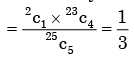QUESTION: 18

If probability density functions of a random variable X is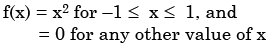Then, the percentage probability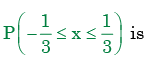Solution: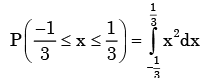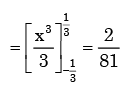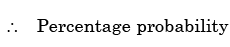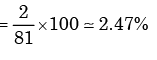QUESTION: 19

Two coins are simultaneously tossed. The probability of two heads simultaneously appearing is

Solution:

Probability of two head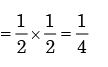QUESTION: 20

A fair coin is tossed three times in succession. If the first toss produces a head, then the probability of getting exactly two heads in three tosses is

Solution:

After first head in first toss, probability of tails in 2nd and 3rd toss =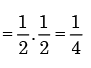∴Probability of exactly two heads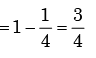QUESTION: 21

A box contains 4 white balls and 3 red balls. In succession, two balls are randomly selected and removed from the box. Given that the first removed ball is white, the probability that the second removed ball is red is

Solution:

After first ball is drawn white then sample space has 4 + 3 – 1 = 6 balls.  Probability of second ball is red without replacement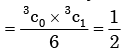QUESTION: 22

Consider a Gaussian distributed radom variable with zero mean and standard deviation σ. The value of its cumulative distribution function at the origin will be

Solution:

Gaussian distributed random variable is commonly known as normal distribution. The distribution is symmetrically distributed about mean and area under the curve is unity. Thus, for half the curve the area will be 0.5 (half of unity) since the curve is symmetric about zero here.

QUESTION: 23

The probability that there are 53 Sundays in a randomly chosen leap year is

Solution:

No. of days in a leap year are 366 days. In which there  are 52 complete weeks and 2 days  extra.

This 2 days may be of following combination.

1. Sunday & Monday

2. Monday & Tuesday

3. Tuesday & Wednesday

4. Wednesday & Thursday

5. Thursday & Friday

6. Friday & Saturday

7. Saturday & Sunday

There are two combination of Sunday in (1.) and (7).

∴ Re quired probability

=2/7

QUESTION: 24

Two dices are rolled simultaneously. The probability that the sum of digits on the top surface of the two dices is even, is

Solution:

Here sample space S= 6 × 6 = 36

Total no. of way in which sum of digits on the top surface of the two dice is is  even is  18.

∴ The require probability = 0.5

QUESTION: 25

The function y = sin φ, (φ > 0) is approximated as y = φ, where φ is in radian. The maximum value of φ for which the error due to the approximation is with in ±2% is

Solution: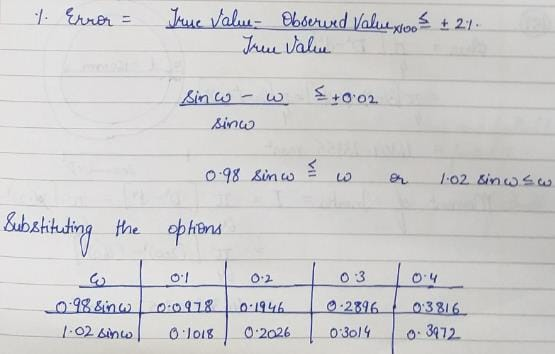QUESTION: 26

Aishwarya studies either computer science or mathematics everyday. if the studies computer science on a day, then the probability that she studies mathematics the next day is 0.6. If she studies mathematics on a day, then the probability that she studies computer science the next day is 0.4. Given that Aishwarya studies computer science on Monday, what is the probability that she studies computer science on Wednesday?

Solution:

Let C denote computes science study and M denotes maths study.

P(C on monday and C on wednesday)

= p(C on monday, M on tuesday and C on wednesday)

+ p(C on monday, C on tuesday and C on wednesday)

= 1 × 0.6 × 0.4 + 1 × 0.4 × 0.4

= 0.24 + 0.16

= 0.40

QUESTION: 27

An examination paper has 150 multiple-choice questions of one mark each, with each question having four choices. Each incorrect answer fetches – 0.25 mark. Suppose 1000 students choose all their answers randomly with uniform probability. The sum total of the expected marks obtained all these students is

Solution:

Let the marks obtained per question be a random variable X. It’s probability  distribution table is given below: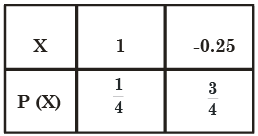Expected mark per question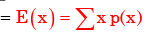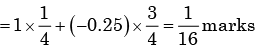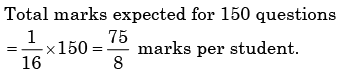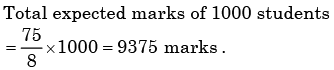QUESTION: 28

Let f(x) be the continuous probability density function of a random variable X. The probability that a < X ≤ b, is

Solution: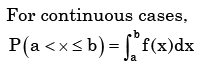QUESTION: 29

Let P(E) denote the probability of the even E. Given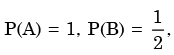the values of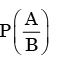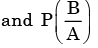respectively are

Solution: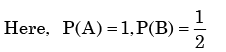We need to find the conditional probability of two given events without being told about P(AB). Also it is not mentioned that they are independent events. But since P(A)=1, it means that A covers the complete sample.

So, P(AB)=P(B)=1/2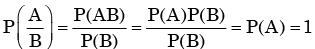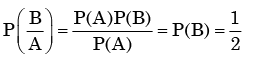QUESTION: 30

For each element is a set of size 2n, an unbiased coin is tossed. The 2n coin tossed are independent. An element is chosen if the corresponding coin toss were head. The probability that exactly n elements are chosen is

Solution:

The probability that exactly n elements are chosen

= the probability of getting n heads out of 2n tosses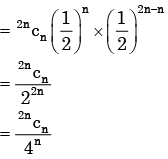Use Code STAYHOME200 and get INR 200 additional OFF Use Coupon Code